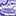# New to Qlik Sense

If you’re new to Qlik Sense, start with this Discussion Board and get up-to-speed quickly.

Announcements
WE ARE LISTENING! New Navigation for Qlik Community, Sept. 26: TELL ME MORE
cancel
Showing results for
Did you mean:Creator II

## Percentage help

Hi,

I have the percentage calculation like below.

Percentage:

Count({<Date={"\$(=Date(Max(Date),'DD/MM/YYYY'))"},Priority={'\$(=\$(vMaxYear)&' '&'Plan')'},[Data Sets]={"=len(Trim([Data Sets]))>0"},[Project]={"=len(Trim([Project]))>0"}>}Distinct ID)

/

Count({<Date={"\$(=Date(Max(Date),'DD/MM/YYYY'))"},Priority={'\$(=\$(vMaxYear)&' '&'Plan')'},[Data Sets]={"=len(Trim([Data Sets]))>0"},[Project]={"=len(Trim([Project]))>0"}>}Total Distinct ID)

Output:

Project       Percentage

A                   12%

B                   15%

C                   32%

etc...

Issue:

But when i have filtered Project = A then 12% turns to 100% same like B and C also by filtering invidual project the percentage turns to 100%.

Labels (1)
• ### Qlik Sense